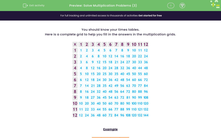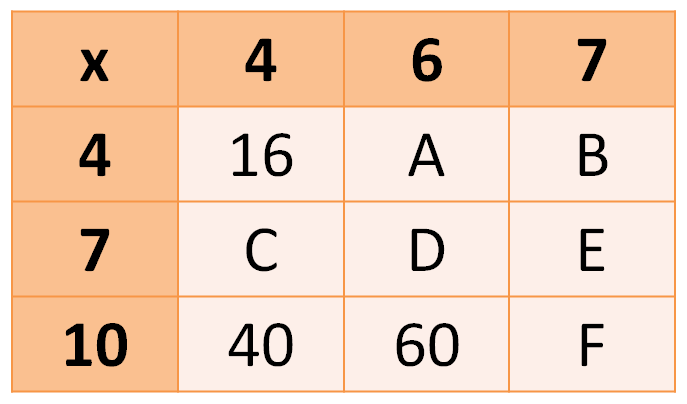# Complete a Multiplication Grid Using Your Times tables

In this worksheet, students will fill in answers to times tables questions on a grid.Key stage:  KS 2

Curriculum topic:   Number: Multiplication and Division

Curriculum subtopic:   Solve Multiplication Problems

Popular topics:   Times Table worksheets

Difficulty level:#### Worksheet Overview

It's a good plan to know your times tables!Example

Can you work out what numbers are represented by the letters in the grid below?This is a times table grid. We can see from the numbers that are filled in, that we need to multiply the number in the column on the left  by the appropriate number in the top row.

4 x 4 = 16

10 x 4 = 40

10 x 6  = 60

So, let's work out what the letters represent.

A = 4 x 6 = 24

B = 4 x 7 = 28

C = 7 x 4 = 28

D = 7 x 6 = 42

E = 7 x 7 = 49

F = 10 x 7 = 70

Does that make sense?Let's have a go at some questions now. If you want to look at this page again, just click on the red help button on the screen.

### What is EdPlace?

We're your National Curriculum aligned online education content provider helping each child succeed in English, maths and science from year 1 to GCSE. With an EdPlace account you’ll be able to track and measure progress, helping each child achieve their best. We build confidence and attainment by personalising each child’s learning at a level that suits them.

Get started